# Non Verbal Reasoning - Figure Matrix

### Exercise :: Figure Matrix - Section 2

In each of the following questions, find out which of the answer figures (1), (2), (3) and (4) completes the figure matrix ?

1.

Select a suitable figure from the four alternatives that would complete the figure matrix.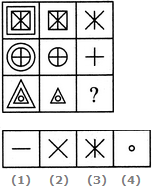A. 1 B. 2 C. 3 D. 4

Explanation:

In each row, the second figure is obtained by removing the outermost element of the first figure and the third figure is obtained by removing the outermost element of the second figure.

2.

Select a suitable figure from the four alternatives that would complete the figure matrix.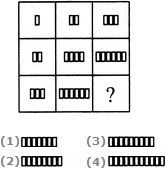A. 1 B. 2 C. 3 D. 4

Explanation:

In each row (as well as in each column), the number of rectangles in the second figure is two times the number of rectangles in the first figure and the number of rectangles in the third figure is three times the number of rectangles in the first figure.

3.

Select a suitable figure from the four alternatives that would complete the figure matrix.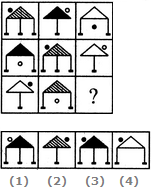A. 1 B. 2 C. 3 D. 4

Explanation:

There are 3 types of shadings in the triangles, 3 types of legs, 3 positions of circles, each of which is used only once in a single row. The circle is shaded in alternate figures.

4.

Select a suitable figure from the four alternatives that would complete the figure matrix.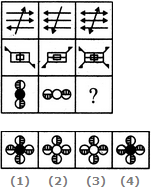A. 1 B. 2 C. 3 D. 4

Explanation:

In each row, the third figure is a combination of the first and the second figure.

5.

Select a suitable figure from the four alternatives that would complete the figure matrix.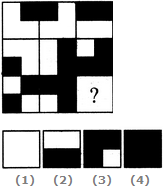A. 1 B. 2 C. 3 D. 4